# LOAM: Lidar Odometry and Mapping in Real-time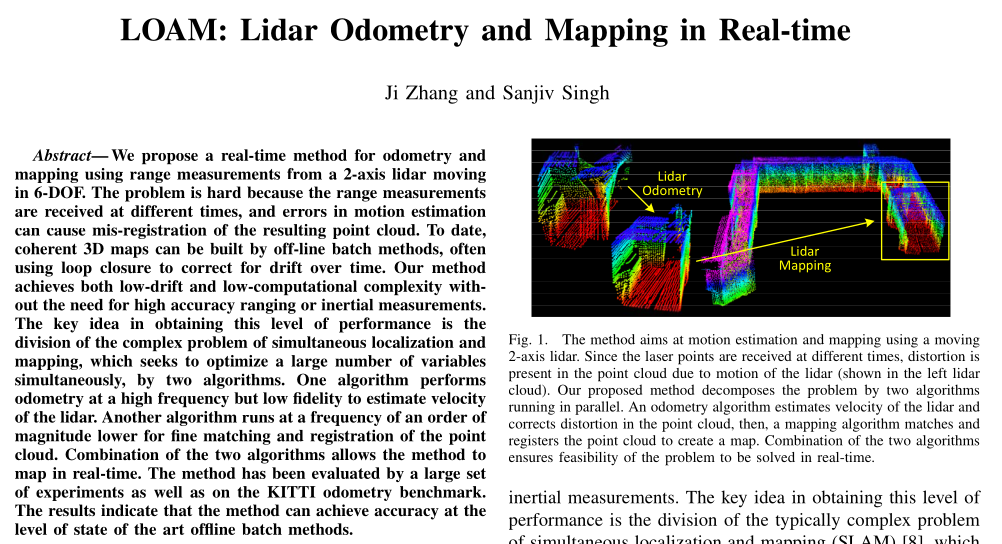# 摘要

• 由于此方法是为了在测程估计中最小化漂移，所以目前不涉及Loop Closure

# 去畸变

• 使用速度来矫正（其他传感器提供速度，或者使用ICP等算法来估计速度）

Barfoot等人使用的一种方法是从激光强度返回中创建视觉目标，并在两帧图像之间匹配视觉上不同的特征，以恢复地面车辆的运动～。其中，文献[18,19]把车辆的运动建模为恒速度模型。

～的方法涉及灰度图像的视觉特征，需要密集的点云，本文提出的方法在笛卡尔空间中提取和匹配几何特征，对云密度要求较低

# 符号约定

• 激光雷达坐标系$$L$$，原点是激光雷达中心，x轴指向左侧，y轴指向上，z轴指向前方。对于此坐标系下的一个点$$i,i \in \mathcal{P}_k$$，可以记为$$X_{(k,i)}^L$$
• 世界坐标系$$W$$，原点是激光雷达的初始位姿，此坐标系下的点，记为$$X_{(k,i)}^W$$

# 系统概述

## 硬件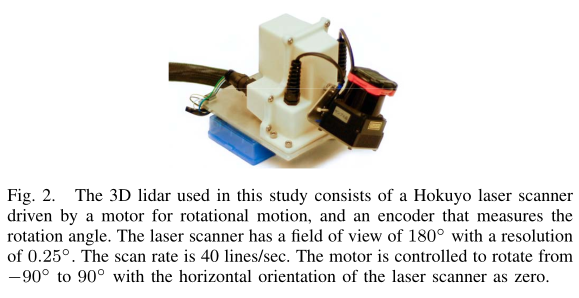## 软件系统• $$\hat{\mathcal{P}}$$作为一帧扫描所得到的点，在每一次扫描中，$$\hat{\mathcal{P}}$$被配准到激光雷达坐标系中
• 然后$$P_k$$使用两种算法来处理：
• 首先是激光里程计，计算两帧扫描来估计激光雷达的相对运动，估计得到的运动反过来矫正$$\mathcal{P}_k$$的畸变，这个算法运行的频率为10Hz。
• 里程计的输出作为建图模块的输入，以1Hz的频率将去畸变后的点云进行匹配和配准到地图上。最后，将两个算法的结果进行整合，以10Hz输出位姿变换矩阵。

# 激光里程计

## 特征提取

• i： 点云$$\mathcal{P}_k$$中的点
• S： 同一帧扫描中与点i连续的点集• 根据计算得到的$$c$$值进行排序，具有最大c值的特征点被选中，作为边缘点
• 具有最小c值的点也选中，作为平面点

• 某个已选中的特征点的附近点
• 与激光束大致平行的平面上的点(如图4(a))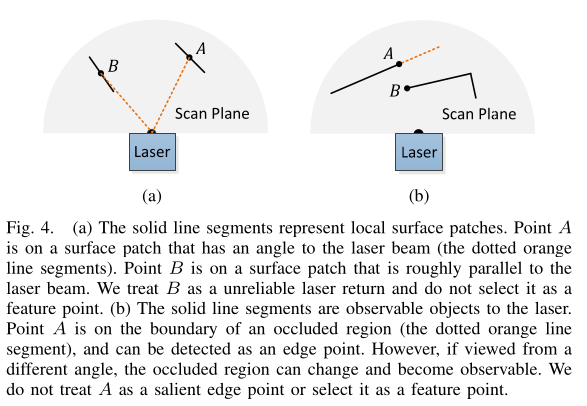• 点集$$\mathcal{S}$$不形成与激光束平行的平面
• 点集$$\mathcal{S}$$没有任何点是(由于激光束方向的gap导致)与点i不连续的，并且比点i更加靠近激光雷达的

！！！则点i才有可能被选中！！！

### 小结

• 选择的边缘点或平面点不超过子区域的最大值
• 候选点i的附近点没有被选中
• 候选点i所在的 局部平面 不能与激光束方向平行，或者是被遮挡区域的边界点

• 黄色：边缘点
• 红色：平面点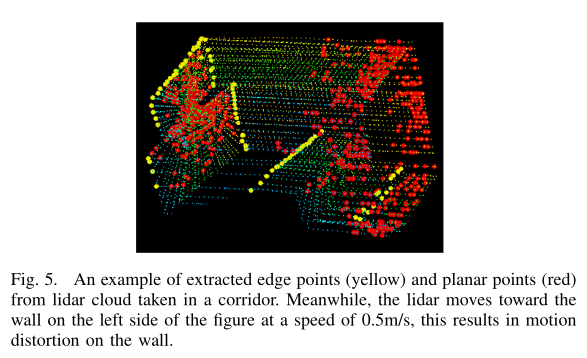## 查找特征点关联

• $$t_k$$：第k帧扫描的起始时间
• $$\bar{\mathcal{P}}_k$$：第k帧扫描重投影到第k+1帧扫描起始时刻的点云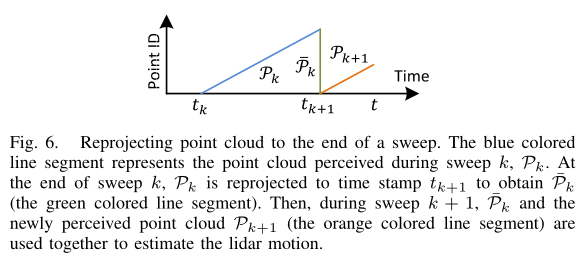• $$\mathcal{E}_{k+1}$$：边缘点集合
• $$\mathcal{H}_{k+1}$$：平面点集合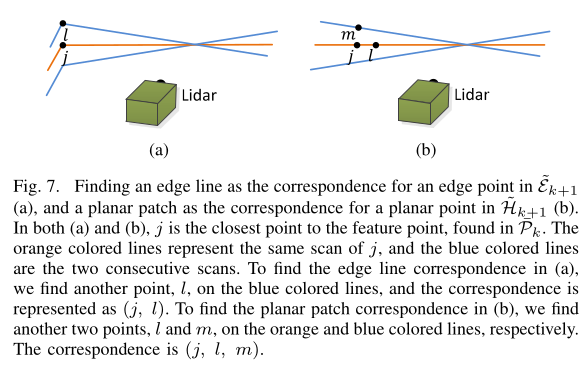### 边缘线点的关联

• $$i$$$$\bar{\mathcal{E}}_{k+1}$$中的点
• $$j$$是在$$\bar{\mathcal{P}}_k$$中与点$$i$$最近邻的点（图中的橙色线上的点）
• $$l$$是两个连续线束扫描中与点$$i$$最近邻的点（图中的上下两条蓝色线束）
• 那么$$(j,l)$$表达了一条关于点$$i$$的边缘线

$$(j,l)$$形成了点i的关联，为了确认点$$j$$$$l$$都是边缘点，我们基于平滑度计算公式来对局部平面的平滑度进行检查。在这里，特别要求$$j$$$$l$$是来自不同线束的扫描，原因是考虑到一线束的扫描中，对于同一个边缘线不能超过1个点。（只有一个例外，即边缘线在扫描平面上，在这种情况下，边缘线会退化为扫描平面上的一条直线，线上的特征点不应该被首先提取）

### 平面点的关联

• $$i$$$$\bar{\mathcal{H}}_{k+1}$$中的点
• $$j$$：在$$\bar{\mathcal{P}}_{k}$$中关于点$$i$$的最近邻点
• $$l$$：在与点$$j$$的同一线圈扫描中，另一个与点$$i$$的最近邻点
• $$m$$：在与点$$j$$的相邻两线圈扫描中，与点$$i$$的最近邻点

1. $$\bar{\mathcal{P}}_{k}$$中查找关于点$$i$$的最近邻点，记为$$j$$
2. 在与点$$j$$的同一线圈中，另一个与$$i$$最近邻的点，记为$$l$$
3. 在与点$$j$$的相邻两线圈扫描中，找到与点$$i$$的最近邻的点，记为$$m$$

### 特征距离表达式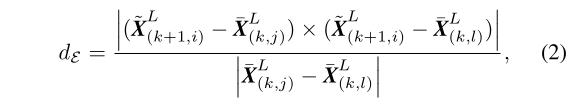• $$\bar{X}_{k+1,i}^L$$是点$$i$$在{L}中的坐标
• $$\bar{X}_{k,j}^L$$是点$$j$$在{L}中的坐标
• $$\bar{X}_{k,l}^L$$是点$$l$$在{L}中的坐标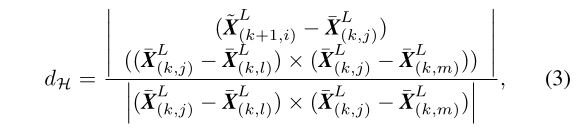• $$\bar{X}_{k,m}^L$$是点$$m$$在{L}中的坐标

## 运动估计

• $$t$$: 当前时刻时间戳
• $$t_{k+1}$$: 在第k+1帧扫描的起始时刻
• $$T_{k+1}^L$$: 在时间段$$[t_{k+1},t]$$之间的激光雷达位姿变换$$T_{k+1}^L=[t_x,t_y,t_z,\theta_x,\theta_y,\theta_z]^T$$(旋转部分遵循右手系)

• $$t_i$$为接收到点$$i$$的对应时刻$$(t_i \in [t_{k+1},t])$$
• $$T_{(k+1,i)}^L$$为时间段$$[t_{k+1},t_i]$$之间的激光雷达位姿变换 ===>$$t_i$$时刻激光雷达坐标系转换到$$t_{k+1}$$时刻的激光雷达坐标系的变换• $$\mathcal{E}_{k+1}$$$$\mathcal{H}_{k+1}$$分别是从$$\mathcal{P}_{k+1}$$提取出来的边缘点和平面点集合
• $$\tilde{\mathcal{E}}_{k+1}$$$$\tilde{\mathcal{H}}_{k+1}$$是将上面的点集重投影回在第$$k+1$$帧扫描的起始时刻的点集

• $$\mathcal{E}_{k+1}$$$$\tilde{\mathcal{E}}_{k+1}$$
• $$\mathcal{P}_{k+1}$$$$\tilde{\mathcal{H}}_{k+1}$$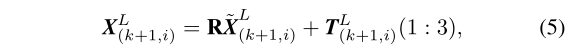• $$X_{(k+1,i)}^L$$是点$$i$$投影到$$t_{k+1}$$时刻下的激光雷达坐标系的坐标
• $$T_{(k+1,i)}^L(1:3)$$表示从$$t_i$$时刻激光雷达坐标系转换到$$t_{k+1}$$时刻的激光雷达坐标系的平移量变换
• $$R$$是使用罗德里格斯公式得到的旋转矩阵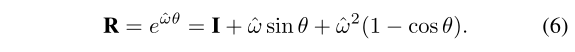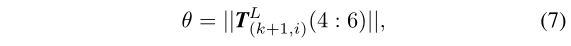$$w$$是单位向量，表示旋转的方向，$$\hat{w}$$是关于向量$$w$$的反对称矩阵: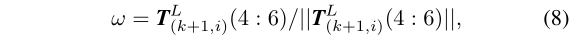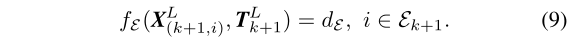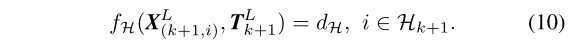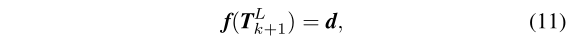• $$f()$$中的每一行与一个特征点相关联
• $$d$$包含了对应的特征关联距离

$J=\frac{\partial f}{\partial T_{k+1}^L}$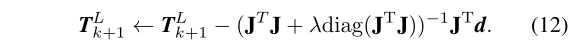$(J^TJ+\lambda)dx=J^Td$

• $$\lambda$$是LM算法的参数

### 小结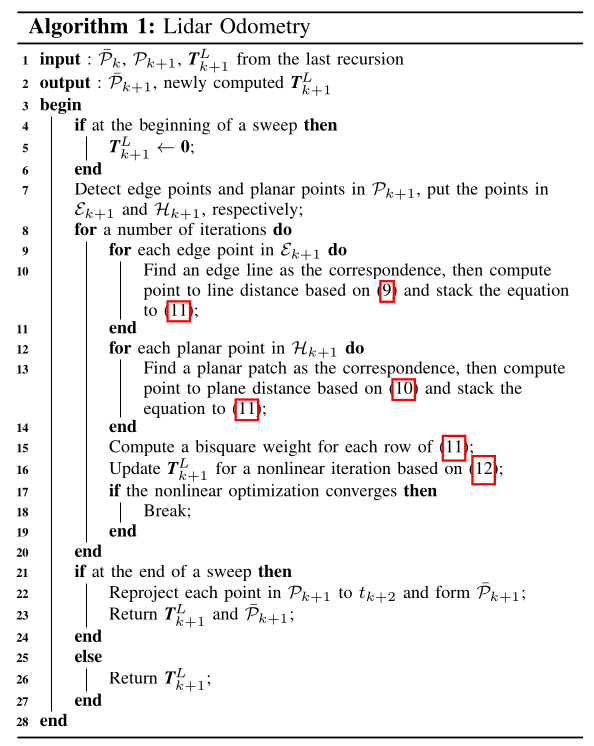# 建图(LIDAR MAPPING)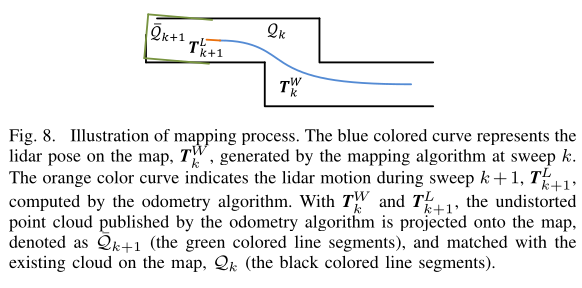• $$Q_k$$: 地图上的点云，是前k帧扫描的累积形成的
• $$T_k^W$$: 在第k帧扫描结束时刻（也是$$t_{k+1}$$），雷达在点云地图上的位姿